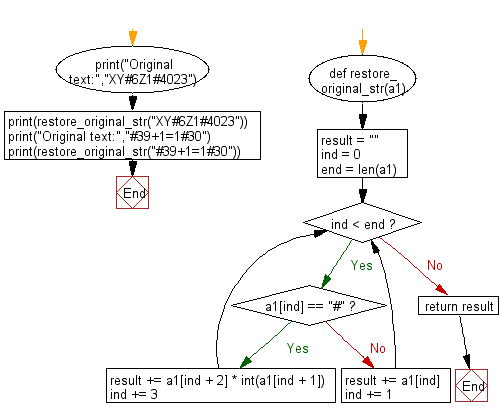﻿ Python: Restore the original string by entering the compressed string with specified rule - w3resource# Python: Restore the original string by entering the compressed string with specified rule

## Python Basic - 1: Exercise-58 with Solution

When character are consecutive in a string , it is possible to shorten the character string by replacing the character with a certain rule. For example, in the case of the character string YYYYY, if it is expressed as # 5 Y, it is compressed by one character.

Write a Python program to restore the original string by entering the compressed string with this rule. However, the # character does not appear in the restored character string.

Note: The original sentences are uppercase letters, lowercase letters, numbers, symbols, less than 100 letters, and consecutive letters are not more than 9 letters.

Input:
The restored character string for each character on one line.
Original text: XY#6Z1#4023
XYZZZZZZ1000023
Original text: #39+1=1#30
999+1=1000

Sample Solution:

Python Code:

``````def restore_original_str(a1):
result = ""
ind = 0
end = len(a1)
while ind < end:
if a1[ind] == "#":
result += a1[ind + 2] * int(a1[ind + 1])
ind += 3
else:
result += a1[ind]
ind += 1
return result
print("Original text:","XY#6Z1#4023")
print(restore_original_str("XY#6Z1#4023"))
print("Original text:","#39+1=1#30")
print(restore_original_str("#39+1=1#30"))
``````

Sample Output:

```Original text: XY#6Z1#4023
XYZZZZZZ1000023
Original text: #39+1=1#30
999+1=1000
```

Pictorial Presentation:Flowchart:Python Code Editor:

Have another way to solve this solution? Contribute your code (and comments) through Disqus.

What is the difficulty level of this exercise?

Test your Programming skills with w3resource's quiz.

﻿

## Python: Tips of the Day

Iterating over dictionaries using 'for' loops:

I am a bit puzzled by the following code: d = {'x': 1, 'y': 2, 'z': 3} for key in d: print key, 'corresponds to', d[key] What I don't understand is the key portion. How does Python recognize ...

key is just a variable name.

```for key in d:
```

For Python 3.x:

```for key, value in d.items():
```

For Python 2.x:

```for key, value in d.iteritems():
```

To test for yourself, change the word key to poop.

In Python 3.x, iteritems() was replaced with simply items(), which returns a set-like view backed by the dict, like iteritems() but even better. This is also available in 2.7 as viewitems().

The operation items() will work for both 2 and 3, but in 2 it will return a list of the dictionary's (key, value) pairs, which will not reflect changes to the dict that happen after the items() call. If you want the 2.x behavior in 3.x, you can call list(d.items()).

Ref: https://bit.ly/37dm0Qo